# Free video lessons for Foundation to Grade 8 students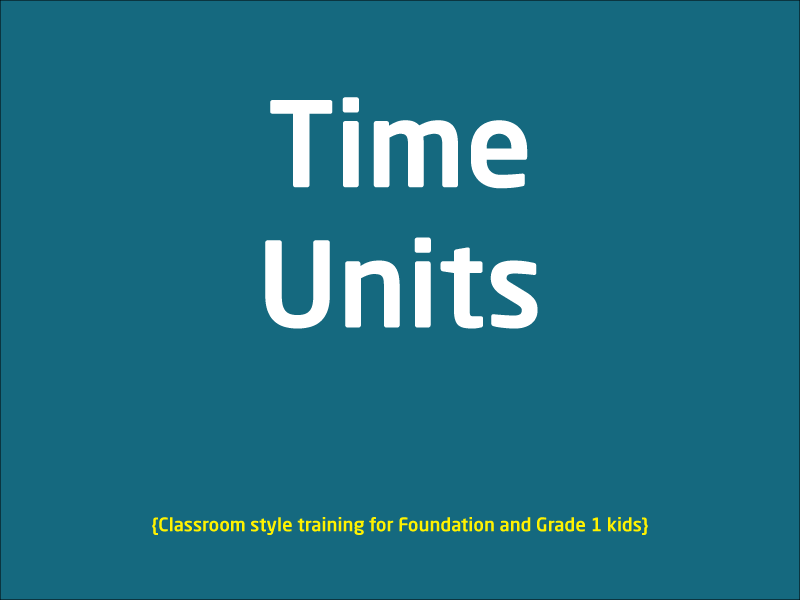### Time units

This Video lesson is all about time units. Tutor will explain time units such as seconds, minutes, hours, days, weeks, months and up to a century. This is a classroom style lesson ...

1 videos
Basic difficulty
Foundation/Maths
0 0 0### Seasons

Learn about seasons in this classroom style video lesson. Several examples are provided to cover all 4 seasons i.e. Summer, Autumn, Winter and Spring.

1 videos
Basic difficulty
Foundation/English
0 0 0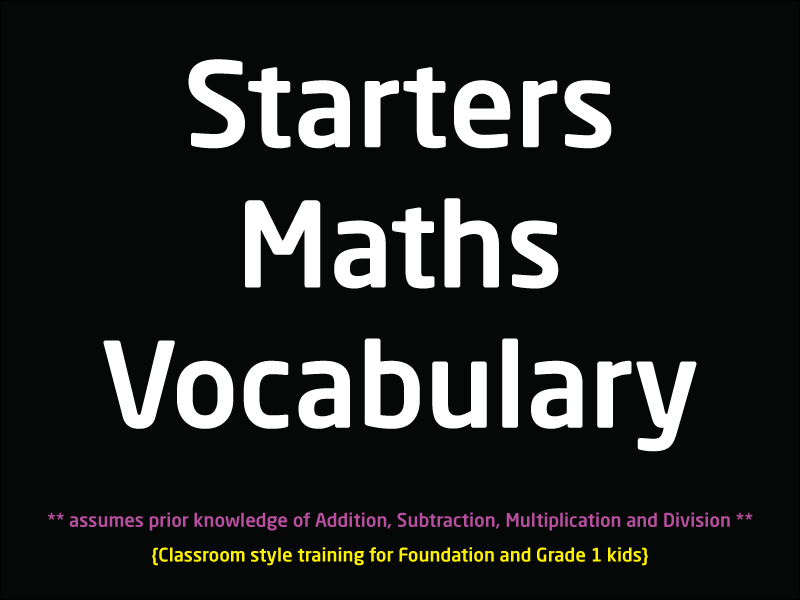### Basic Maths Vocabulary for Foundation and Grade 1 kids

This video lesson covers some core mathematical operation sign and explains what they are and what are they called. e.g. + is called a plus sign and is used in addition/sum/adding,...

1 videos
Basic difficulty
Foundation/Maths
0 0 0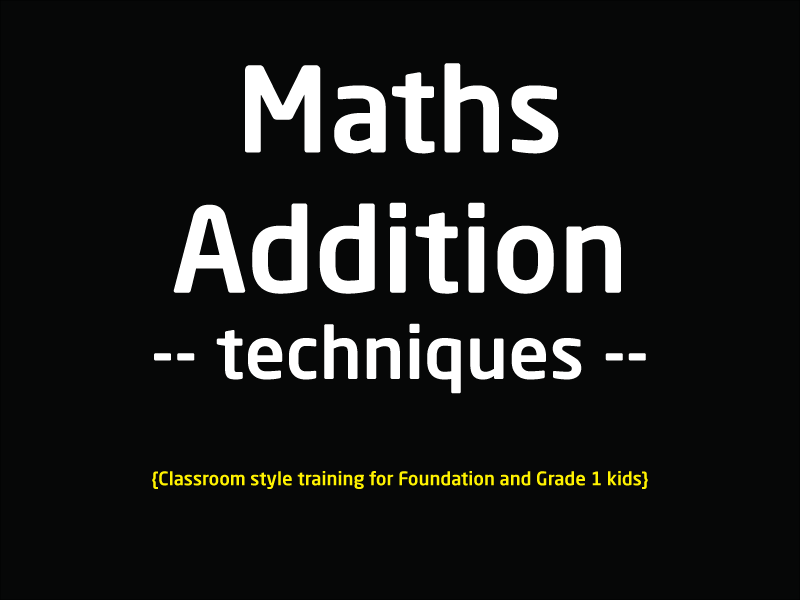This lesson is for kids who are starting off with addition, tutor explains different techniques kids can use to add number that produce a result of number 10 or less. There are sev...

1 videos
Basic difficulty
Foundation/Maths
0 0 0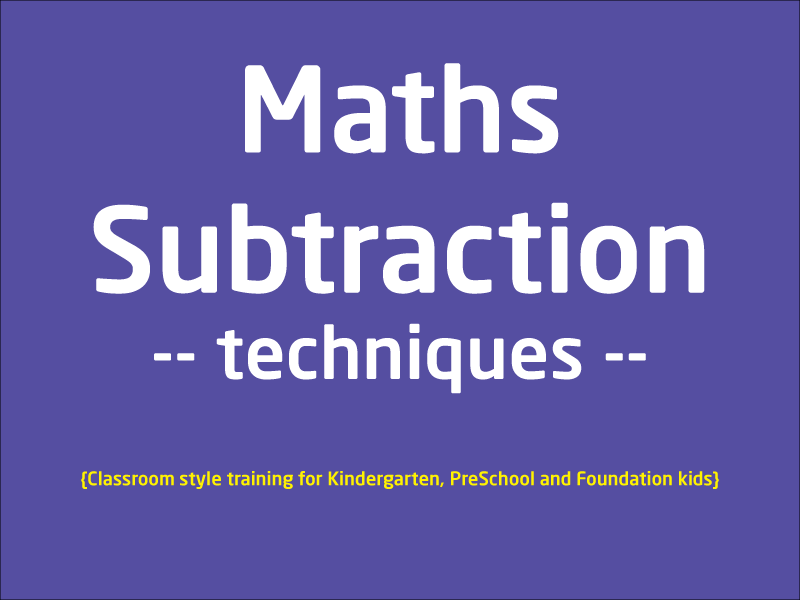### Learn Subtraction

This video lesson covers Subtraction which is kinda opposite to addition. In this lesson kids will learn techniques that will assist them to start with subtraction. Kids will feel ...

1 videos
Basic difficulty
Foundation/Maths
0 0 0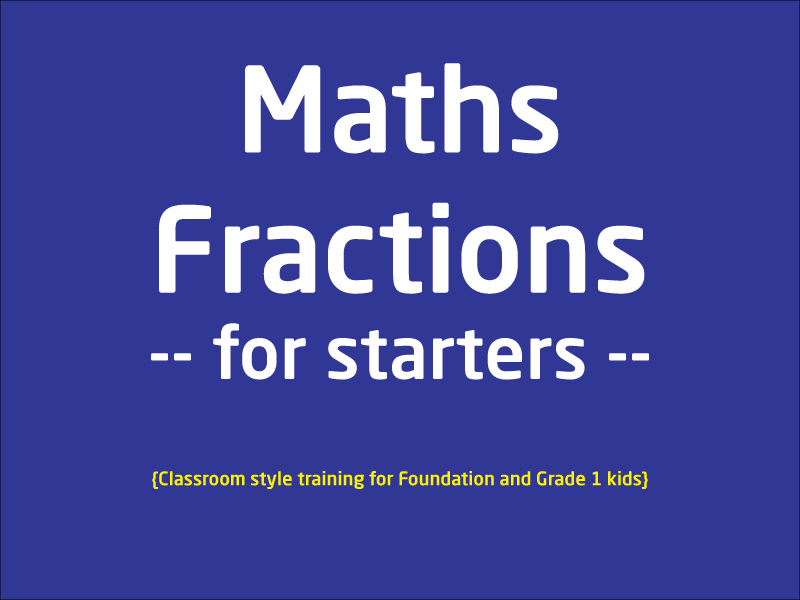### Fractions for Starters

This lesson is for kids starting off to learn fractions. Tutor discuss different kinds of fractions, for example, one-half (1/2), one-third (1/3) or one-fourth (1/4) etc. This is a...

1 videos
Basic difficulty
Foundation/Maths
0 0 0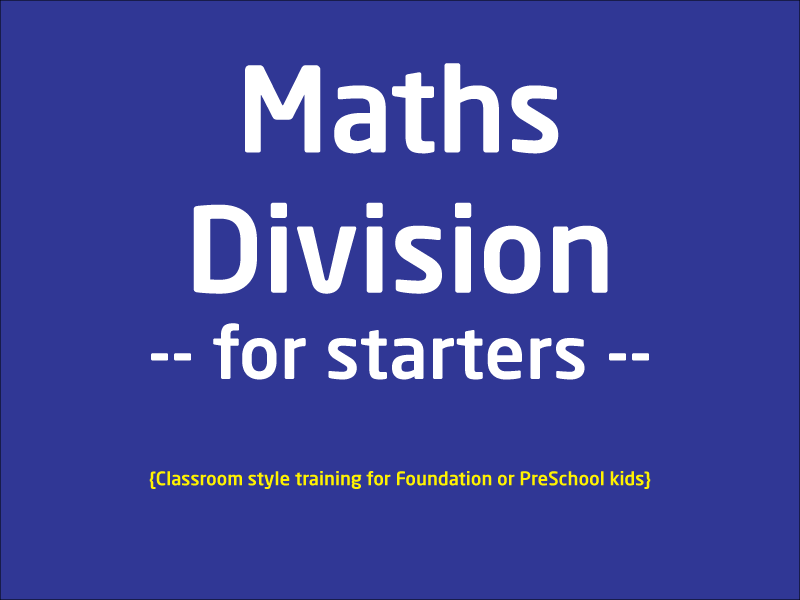### Maths division - The Basics

This lesson is a half an hour lesson explaining kids how to divide basically. This lesson does not cover remainder because that would make things too complicated for children. Rema...

1 videos
Basic difficulty
Foundation/Maths
0 0 0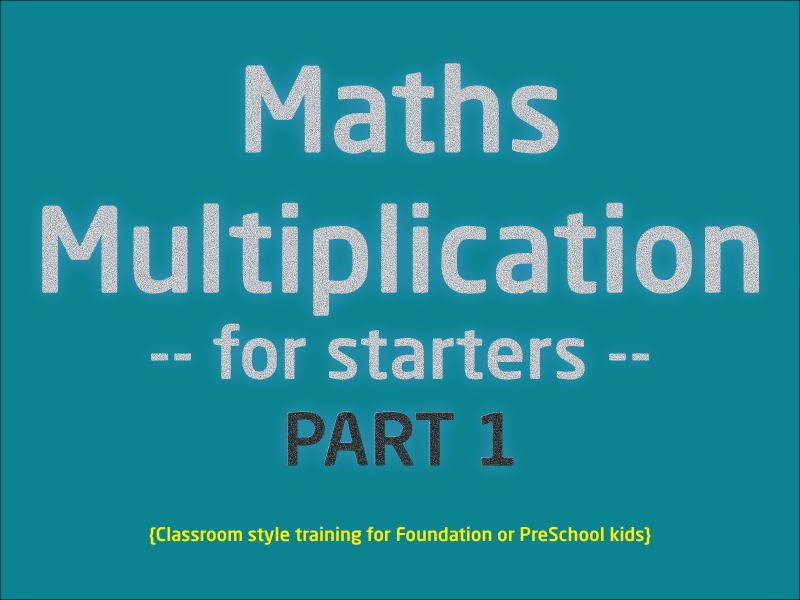### Multiplication for Starters

This lesson is split into 2 partsPart 1 discuss about the basics of multiplication and how new students should approach it.Part 2 shows visual examples plus multiplication table fo...

2 videos
Basic difficulty
Foundation/Maths
0 0 0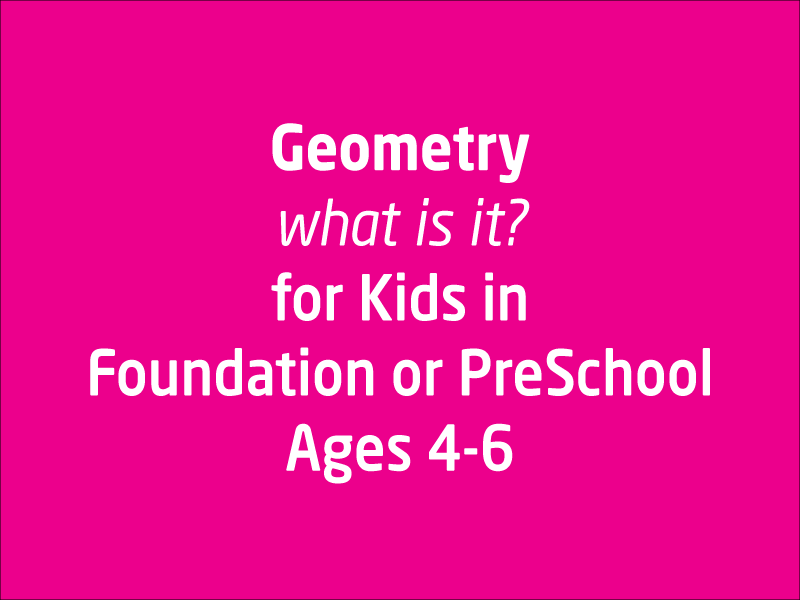### Geometry for Foundation and PreSchoolers

This lesson is not about understanding each and every concept covered in Geometry, because that's a vast and big subject in itself. However for little ones it gives them an opp...

1 videos
Basic difficulty
Foundation/Maths
0 0 0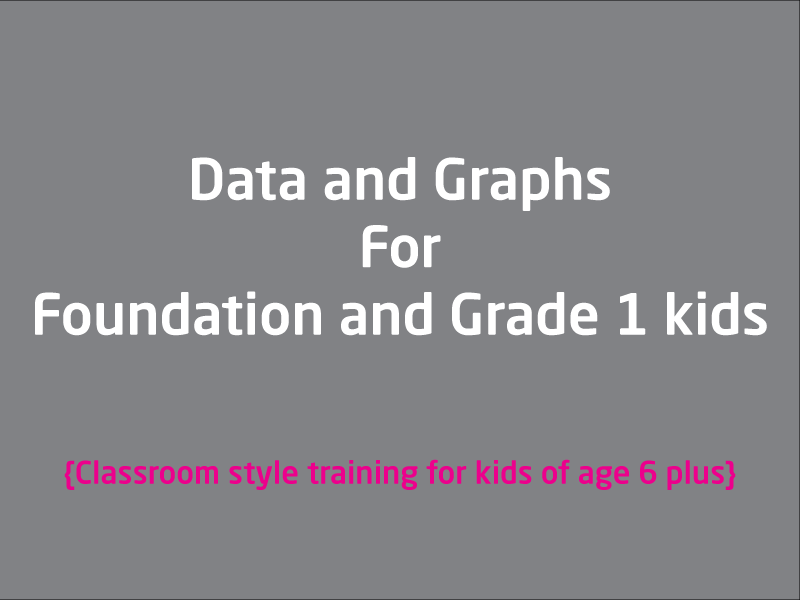### Introduction to Data and Graph

For kids 6 plus, this lesson can be very useful if they are just starting off to understand what Data is and What graph means. This is a live recorded classroom style lesson, send ...

1 videos
Basic difficulty
Foundation/Maths
0 0 0